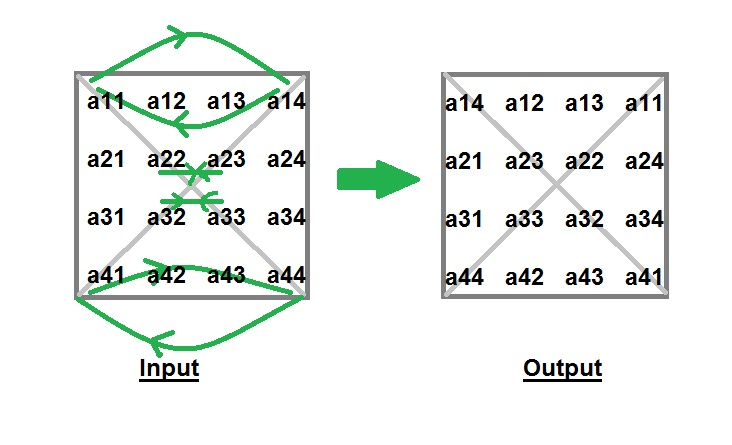# Python Program to Interchange Diagonals of Matrix

• Last Updated : 24 May, 2022

Given a square matrix of order n*n, you have to interchange the elements of both diagonals.
Examples :

```Input : matrix[][] = {1, 2, 3,
4, 5, 6,
7, 8, 9}
Output : matrix[][] = {3, 2, 1,
4, 5, 6,
9, 8, 7}

Input : matrix[][] = {4,  2,  3,  1,
5,  7,  6,  8,
9, 11, 10, 12,
16, 14, 15, 13}
Output : matrix[][] = {1,  2,  3,  4,
5,  6,  7,  8,
9, 10, 11, 12,
11, 14, 15, 16}```

Explanation : Idea behind interchanging diagonals of a square matrix is simple. Iterate from 0 to n-1 and for each iteration you have to swap a[i][i] and a[i][n-i-1].## Python3

 `# Python program to interchange``# the diagonals of matrix``N ``=` `3``;` `# Function to interchange diagonals``def` `interchangeDiagonals(array):` `    ``# swap elements of diagonal``    ``for` `i ``in` `range``(N):``        ``if` `(i !``=` `N ``/` `2``):``            ``temp ``=` `array[i][i];``            ``array[i][i] ``=` `array[i][N ``-` `i ``-` `1``];``            ``array[i][N ``-` `i ``-` `1``] ``=` `temp;``        ` `    ``for` `i ``in` `range``(N):``        ``for` `j ``in` `range``(N):``            ``print``(array[i][j], end ``=` `" "``);``        ``print``();` `# Driver Code``if` `__name__ ``=``=` `'__main__'``:``    ``array ``=` `[ ``4``, ``5``, ``6` `],[ ``1``, ``2``, ``3` `],[ ``7``, ``8``, ``9` `];``    ``interchangeDiagonals(array);``    ` `# This code is contributed by Rajput-Ji`

Output:

``` 6 5 4
1 2 3
9 8 7```

Time Complexity: O(N*N), as we are using nested loops for traversing the matrix.

Auxiliary Space: O(1), as we are not using any extra space.

Please refer complete article on Program to Interchange Diagonals of Matrix for more details!

My Personal Notes arrow_drop_up